Anúncio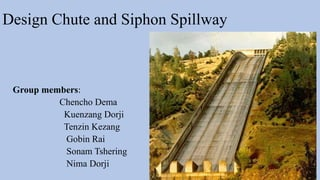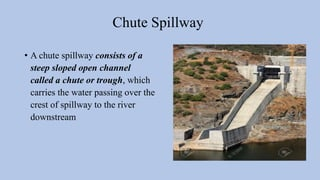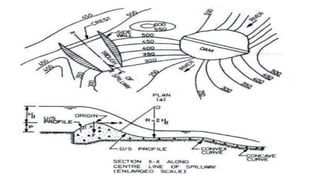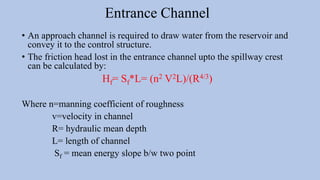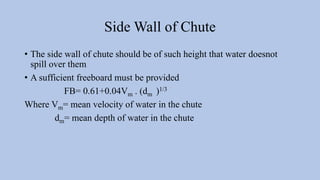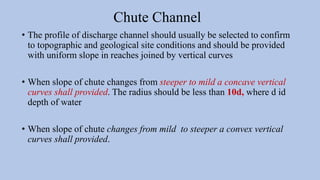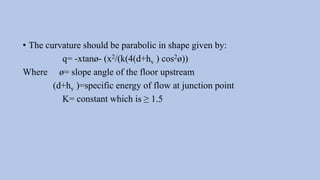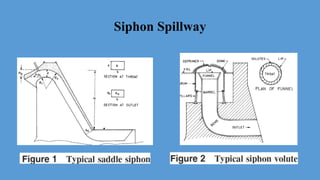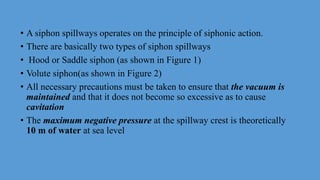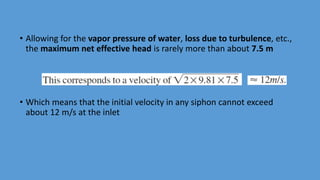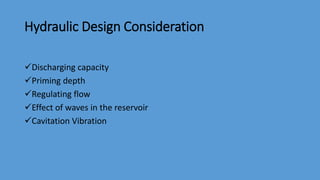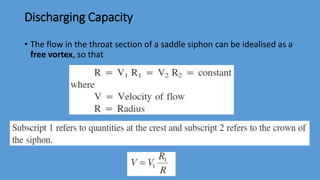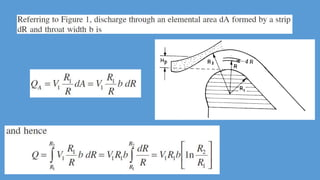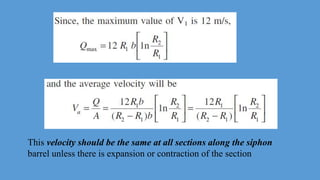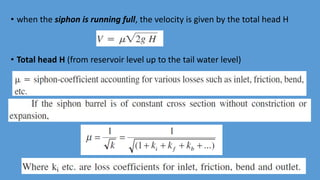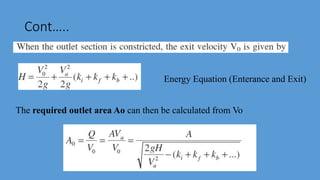1 de 17
Anúncio

### design of chute and syphonspillway

1. Design Chute and Siphon Spillway Group members: Chencho Dema Kuenzang Dorji Tenzin Kezang Gobin Rai Sonam Tshering Nima Dorji
2. Chute Spillway • A chute spillway consists of a steep sloped open channel called a chute or trough, which carries the water passing over the crest of spillway to the river downstream
3. Entrance Channel • An approach channel is required to draw water from the reservoir and convey it to the control structure. • The friction head lost in the entrance channel upto the spillway crest can be calculated by: Hf= Sf*L= (n2 V2L)/(R4/3) Where n=manning coefficient of roughness v=velocity in channel R= hydraulic mean depth L= length of channel Sf = mean energy slope b/w two point
4. Side Wall of Chute • The side wall of chute should be of such height that water doesnot spill over them • A sufficient freeboard must be provided FB= 0.61+0.04Vm . (dm )1/3 Where Vm= mean velocity of water in the chute dm= mean depth of water in the chute
5. Chute Channel • The profile of discharge channel should usually be selected to confirm to topographic and geological site conditions and should be provided with uniform slope in reaches joined by vertical curves • When slope of chute changes from steeper to mild a concave vertical curves shall provided. The radius should be less than 10d, where d id depth of water • When slope of chute changes from mild to steeper a convex vertical curves shall provided.
6. • The curvature should be parabolic in shape given by: q= -xtanø- (x2/(k(4(d+hv ) cos2ø)) Where ø= slope angle of the floor upstream (d+hv )=specific energy of flow at junction point K= constant which is ≥ 1.5
7. Siphon Spillway
8. • A siphon spillways operates on the principle of siphonic action. • There are basically two types of siphon spillways • Hood or Saddle siphon (as shown in Figure 1) • Volute siphon(as shown in Figure 2) • All necessary precautions must be taken to ensure that the vacuum is maintained and that it does not become so excessive as to cause cavitation • The maximum negative pressure at the spillway crest is theoretically 10 m of water at sea level
9. • Allowing for the vapor pressure of water, loss due to turbulence, etc., the maximum net effective head is rarely more than about 7.5 m • Which means that the initial velocity in any siphon cannot exceed about 12 m/s at the inlet
10. Hydraulic Design Consideration Discharging capacity Priming depth Regulating flow Effect of waves in the reservoir Cavitation Vibration
11. Discharging Capacity • The flow in the throat section of a saddle siphon can be idealised as a free vortex, so that
12. This velocity should be the same at all sections along the siphon barrel unless there is expansion or contraction of the section
13. • when the siphon is running full, the velocity is given by the total head H • Total head H (from reservoir level up to the tail water level)
14. Cont….. Energy Equation (Enterance and Exit) The required outlet area Ao can then be calculated from Vo
15. THANK YOU
Anúncio